# MVVM

5 Dec 2017 / 14 minutes to read

## AngularJS

Circular Gauge contains AngularJS support. You can add object as well as array object in the Circular Gauge. The two way binding support is given to the pointer value, minimum scale value and maximum scale value.

## Rendering the Circular Gauge

ej-CircularGauge is the control tag in which ej is tag prefix and CircularGauge is the control name.The following code example helps you to render Circular Gauge.

• html
• ``````<!--To Render the Circular gauge-->
<!doctype html>
<html ng-app="syncApp">
<!--Refer the necessary script here-->
<body ng-controller="CircularGauge">
<ej-circulargauge id="CircularGauge1" e-backgroundcolor="transparent" e-value="50"
e-enableanimation="false">
</ej-circulargauge>
<script type="text/javascript">
<!--binding the value to the scope variables in application controller-->
angular.module('syncApp', ['ejangular'])
.controller('CircularGauge', function (\$scope) {
\$scope.number = 50;
\$scope.minimum = 0;
\$scope.maximum = 120;
});
</script>
</body>
</html>``````

Execute the above code to render the output as follows.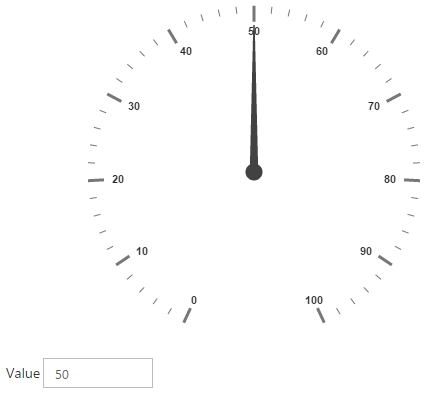Scale is an array object and you can use the inner tag for it. Object in the array collection (i.e. border) is extended with hyphen in the same tag.

Example: e-border-width and e-border-color.

• html
• ``````<!--To Render the Circular gauge-->
<ej-circulargauge id="CircularGauge1">
<!--Adding Scale collection to the Circular gauge-->
<e-scales>
<e-scale e-showRanges="true" e-startAngle="122" e-sweepAngle="296"
e-majorIntervalValue="20" e-minorIntervalValue="10"
e-border-width="0.5">
</e-scale>
</e-scales>
</ej-circulargauge>``````

Execute the above code to render the following output.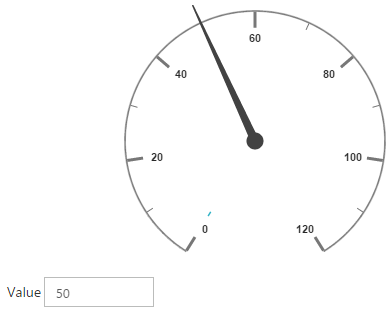Pointer is an array object and you can use the inner tag for it. Object in the array collection (i.e. pointer cap) is extended with hyphen in the same tag.

• html
• ``````<!--To Render the Circular gauge-->
<ej-CircularGauge id="CircularGauge1">
<!--Adding Scale collection to the Circular gauge-->
<e-scales>
<e-scale>
<!--Adding pointer collection to the scale collection-->
<e-pointers>
<e-pointer e-showBackNeedle="true" e-backNeedleLength="20"
e-length="95" e-width="7" e-value="80"
</e-pointer>
</e-pointers>
</e-scale>
</e-scales>
</ej-CircularGauge>``````

Execute the above code to render the output as follows.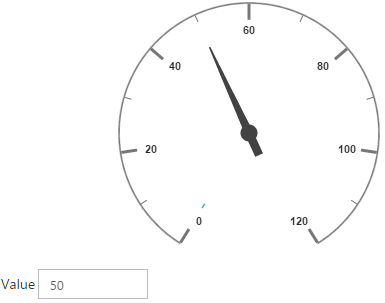Label is also an array object. You can use the inner tag for it.

• html
• ``````<!--To Render the Circular gauge-->
<ej-CircularGauge id="CircularGauge1">
<!--Adding Scale collection to the Circular gauge-->
<e-scales>
<e-scale>
<!--Adding pointer collection to the scale collection-->
<e-pointers>…</e-pointers>
<!--Adding labels collection to the scale collection-->
<e-labels>
<e-label e-color="#8c8c8c">
</e-label>
</e-labels>
</e-scale>
</e-scales>
</ej-CircularGauge>``````

Execute the above code to render the following output.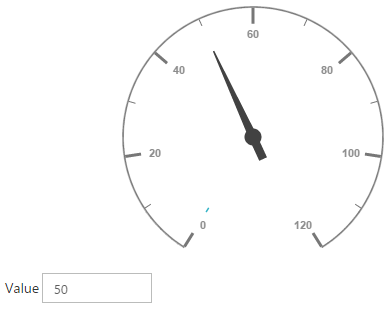Tick is an array object. You can use the inner tag for it.

• html
• ``````<!--To Render the Circular gauge-->
<ej-CircularGauge id="CircularGauge1">
<!--Adding Scale collection to the Circular gauge-->
<e-scales>
<e-scale>
<!--Adding pointer collection to the scale collection-->
<e-pointers>…</e-pointers>
<!--Adding labels collection to the scale collection-->
<e-labels>…</e-labels>
<!--Adding ticks collection to the scale collection-->
<e-ticks>
<e-tick e-type="major" e-distanceFromScale="2" e-height="16"
e-width="1" e-color="#8c8c8c">
</e-tick>
<e-tick e-type="minor" e-distanceFromScale="2" e-height="8"
e-width="1" e-color="#8c8c8c">
</e-tick>
</e-ticks>
</e-scale>
</e-scales>
</ej-CircularGauge>``````

Execute the above code to render the following output.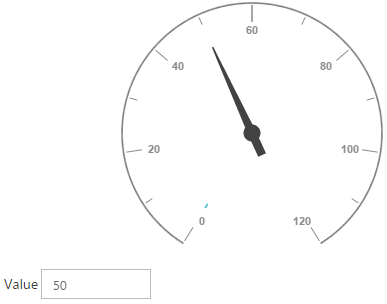Range is an array object. You can use the inner tag for it. Object in the array collection (i.e. border) is extended with hyphen in the same tag.

Example: e-border-color.

• html
• ``````<!--To Render the Circular gauge-->
<ej-circulargauge id="CircularGauge1">
<!--Adding Scale collection to the Circular gauge-->
<e-scales>
<e-scale>
<!--Adding pointer collection to the scale collection-->
<e-pointers>…</e-pointers>
<!--Adding labels collection to the scale collection-->
<e-labels>…</e-labels>
<!--Adding ticks collection to the scale collection-->
<e-ticks>…</e-ticks>
<!--Adding ranges collection to the scale collection-->
<e-ranges>
<e-range e-distanceFromScale="-30" e-startValue="0" e-endValue="70">
</e-range>
<e-range e-distanceFromScale="-30" e-startValue="70"
e-endValue="110" e-backgroundColor="#fc0606"
e-border-color="#fc0606">
</e-range>
<e-range e-distanceFromScale="-30" e-startValue="110"
e-endValue="120" e-backgroundColor="#f5b43f"
e-border-color="#f5b43f">
</e-range>
</e-ranges>
</e-scale>
</e-scales>
</ej-circulargauge>``````

Execute the above code to render the following output.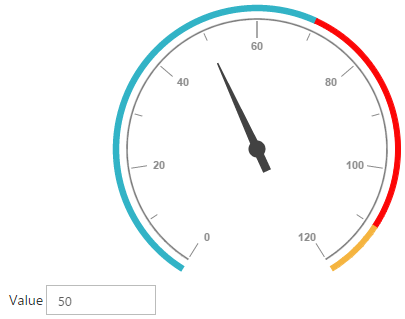## Two Way Binding

Circular Gauge support the two way binding for the property value, minimum and maximum as mentioned earlier. The following code example explains how to achieve the two way binding in Circular Gauge.

• html
• ``````<!doctype html>
<html ng-app="syncApp">
<!--Refer the necessary script here-->
<body ng-controller="CircularGauge">
<div id="linearframe">
<e-scales>
<e-scale e-showRanges="true" e-startAngle="122" e-sweepAngle="296"
<!--binding maximum value using angular JS -->
e-maximum="maximum"
<!--binding minimum value using angular JS -->
e-minimum="minimum"
e-majorIntervalValue="20"
e-minorIntervalValue="10" e-border-width="0.5">
<e-pointers>
<e-pointer e-showBackNeedle="true" e-backNeedleLength="20"
e-length="95" e-width="7"
<!--binding pointer value using angular JS -->
e-value="number"
</e-pointer>
</e-pointers>
<e-labels>
<e-label e-color="#8c8c8c"></e-label>
</e-labels>
<e-ticks>
<e-tick e-type="major" e-distanceFromScale="2" e-height="16"
e-width="1" e-color="#8c8c8c"></e-tick>
<e-tick e-type="minor" e-distanceFromScale="2" e-height="8"
e-width="1" e-color="#8c8c8c"></e-tick>
</e-ticks>
<e-ranges>
<e-range e-distanceFromScale="-30" e-startValue="0" e-endValue="70"></e-range>
<e-range e-distanceFromScale="-30" e-startValue="70"
e-endValue="110" e-backgroundColor="#fc0606"
e-border-color="#fc0606"></e-range>
<e-range e-distanceFromScale="-30" e-startValue="110"
e-endValue="120" e-backgroundColor="#f5b43f"
e-border-color="#f5b43f"></e-range>
</e-ranges>
</e-scale>
</e-scales>
</ej-circulargauge>
</div>
<input type="text" id="txtMax" e-value="number" ej-numerictextbox **ng-model="number"**  e-decimalplaces="2" e-showspinbutton="false" Style="width:110px"/>
<script type="text/javascript">
<!--binding the value to the scope variables in application controller-->
angular.module('syncApp', ['ejangular'])
.controller('CircularGauge', function (\$scope) {
\$scope.number = 50;
\$scope.minimum = 0;
\$scope.maximum = 120;
});
</script>
</body>
</html>``````

Execute the above code to render the following output.